# Introduction to IIR Filters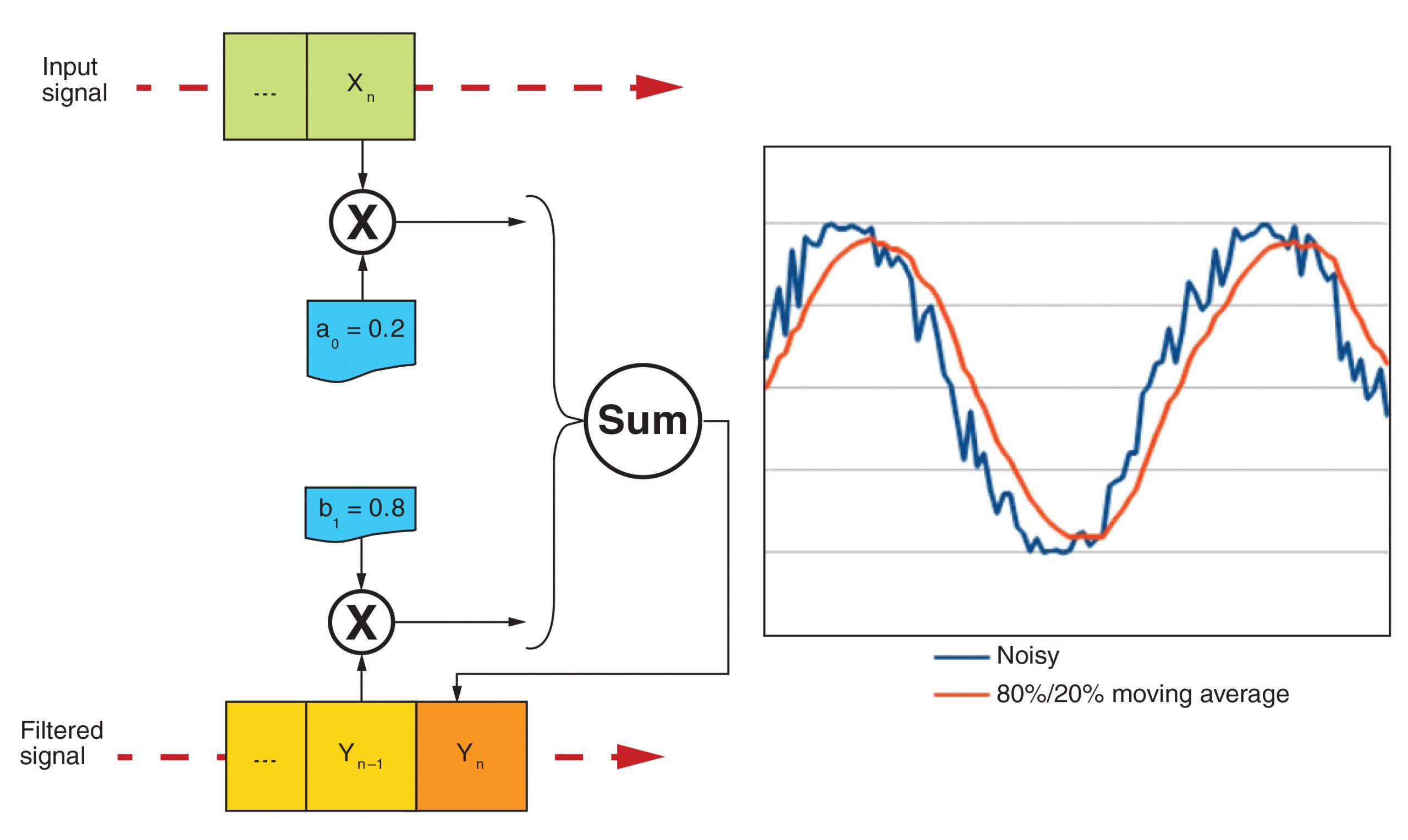Written by

### Infinite impulse response (IIR) filters are feedback systems that use their outputs as inputs. It’s easy to design a simple IIR filter and program it in your application if you know what tools to use. This article uses some simple math to provide the basics on IIR filters and explains the difference and similarities between IIR filters, finite impulse response (FIR) filters, and analog filters.

Hello, all. Welcome back to The Darker Side. I started this column in August 2007 (“Let’s Play with EMI!,” Circuit Cellar, 205). At that time, I thought I would have interesting electronics engineering topics to share with you for a year, maybe two. I am delighted to still be in front of my keyboard five years later. But, it’s thanks to you. Over these five years, the great feedback and e-mail discussions with you, Circuit Cellar readers, have motivated me to continue! Moreover, my article topics are often inspired by readers’ comments.

This month’s article is a good example. My second Darker Side article was about finite impulse response (FIR) digital filters (“No Fear with FIR: Put a FIR Filter to Work,” Circuit Cellar 207, 2007). I received five or six e-mails after it was published. Two of you asked me to write something about the other kind of digital filters—the so-called infinite impulse response (IIR) filters. At the time, I was reluctant. I found it difficult to present IIR filters in a short article, probably because I was not familiar enough with them. Time has passed, and now I will try to fulfill your requests.

One of the difficult things about IIR filters is that they are usually presented through exotic mathematical concepts (e.g., poles and zeros placement, Z-transforms, etc.) While these methods are invaluable, I want to explain the basics of IIR filters using simple words, math, and electronic concepts. Relax and enjoy. I think I found a solution. In this article, I will use the much-loved “soldering iron language” to show that IIR filters are similar to classic analog filters.

##### FIR & IIR

Let’s start with the basics. A digital filter is a piece of software, firmware, or logic circuit that takes a digital data flow as an input and provides a filtered version of this signal on its output. As we live in a digital world, this data flow is a discrete-time signal (i.e., its successive values represent the signal value at discrete time steps). There’s nothing new here. However, as an efficient time machine has yet to be invented, at a given time step, a real-time digital filter must calculate the next output value based only on older values. There are two ways to do this. The simplest one uses the FIR filter presented in my 207 article. As indicated by the article title, this type of FIR filter has a finite “memory” over time. In practical words, a FIR filter takes the N previous input samples and uses them to calculate the next output value (see Figure 1a). For example, you can build a moving average filter by calculating the average of the last 10 input values. Its algorithm would look like what is shown in Figure 2.

That’s a kind of FIR filter, as the new output value is calculated as a linear combination of a fixed number of previous input values, here 10. An IIR filter uses a slightly more complex, but more powerful approach. Rather than just using the previous input values, an IIR filter calculates the next output value based on the previous input values and the previously calculated output values (see Figure 1b). So, an IIR filter is a feedback system. Its output is used as an input. As a consequence, an input change may influence the output for a long, theoretically infinite, time. Let’s go back to the example of a moving average filter. Instead of calculating the last 10 input values’ average, which is a FIR, you could calculate a moving average by adjusting an already calculated average based on the next input value. This will give you a basic IIR. The corresponding algorithm is shown in Figure 3.

Figure 4 shows this type of filter on a noisy sine signal with x = 0.2. Simply increase the x factor for a lower filtering factor, or decrease it to have a stronger filter. In this basic example, the output signal is calculated with only the last input and output values, multiplying each of them by a given constant and summing the results to get the new output value. But think about it, you could build more complex filters by adding more parameters. There is nothing to prevent you from using the same method but with a larger number of previous input and output values, not just the last one of each. This provides an IIR filter’s generic form (see Figure 5). The principle is still the same simple algorithm. Store the N previous input samples and the previously calculated output values in a circular buffer, multiply each buffer entry with a corresponding numerical constant at each time step, and sum them all to get the new output value.

If you think about it, a FIR is a specific type of IIR, with all feedback coefficients set to zero. An IIR must be more powerful than a FIR, but what are IIR filters’ advantages compared to FIR filters? Thanks to the feedback mechanism, the same filtering performance could be achieved with much fewer calculations. Look again at the moving average example. The FIR variant required 10 multiplications per sample, where the IIR version needs only two. So, an IIR filter costs far less than a FIR in terms of instructions per second or logic gates. This must be a disadvantage, right? You bet. First, a FIR filter can easily implement virtually any frequency response you wish, where designing an IIR for something other than classical filters (e.g., low pass, bandpass, high pass, or notch) is a real challenge. That’s a limitation, even if this satisfies 99% of the applications. Second, it’s difficult to find the IIRs coefficients’ values. This is tricky, especially as an IIR filter may easily diverge or provide random results with improper coefficients. For example, you can check what would provide the “moving average” algorithm if the sum of both coefficients was accidentally a little higher than one. The output value will be infinite very soon. Last, as an IIR filter uses feedback, any error (e.g., truncation errors), may accumulate over time. This makes the designer’s life difficult.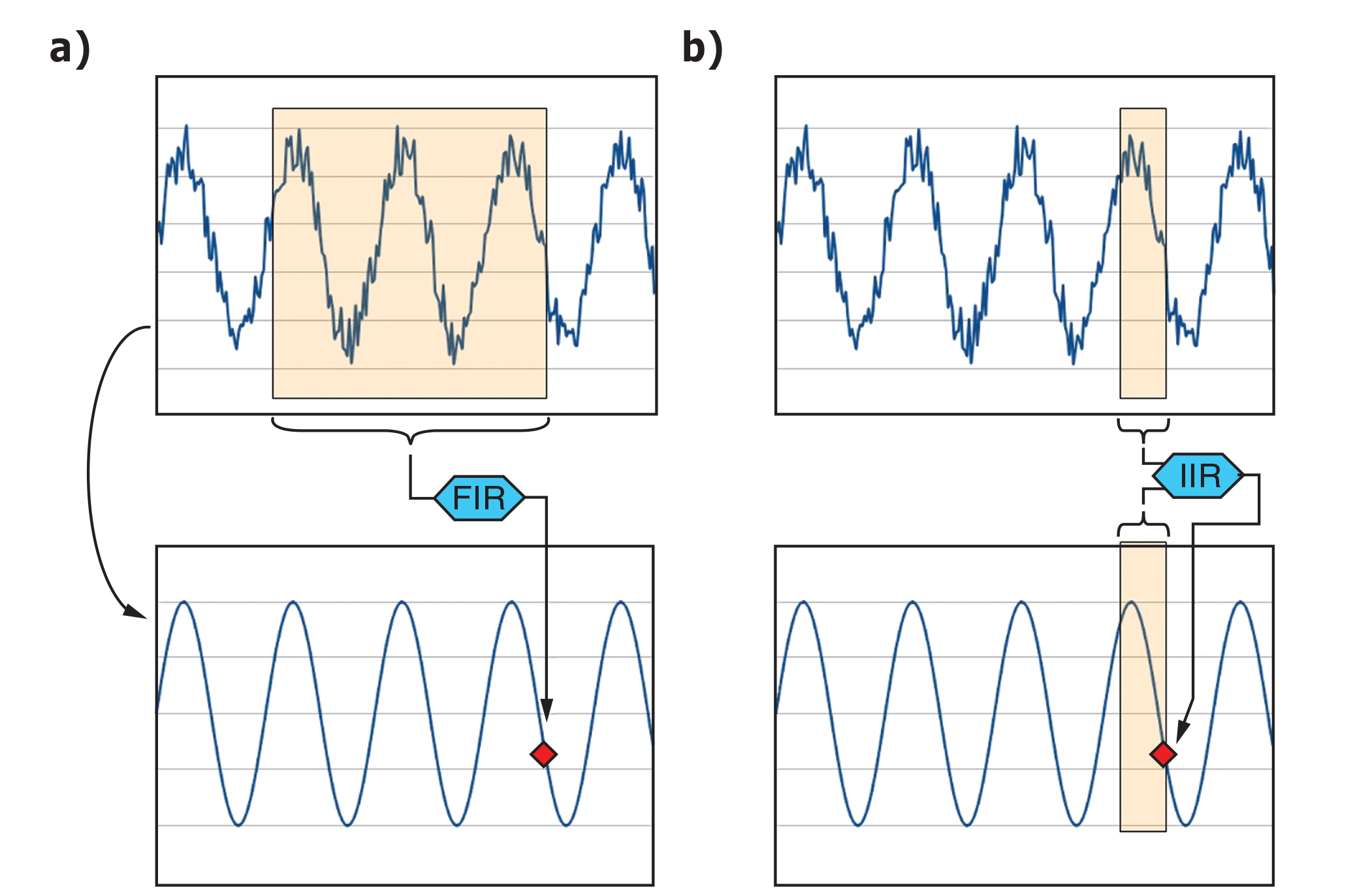Figure 1—A FIR filter calculates the output signal’s next value based on the input signal’s fixed-duration segment. In contrast, an IIR filter uses the input signal’s previous values and previously calculated output values. This introduces a kind of feedback and enables the use of smaller data sets for the same performance.Figure 2—Produce this algorithm by building a moving average filter by calculating the average of the last 10 input values.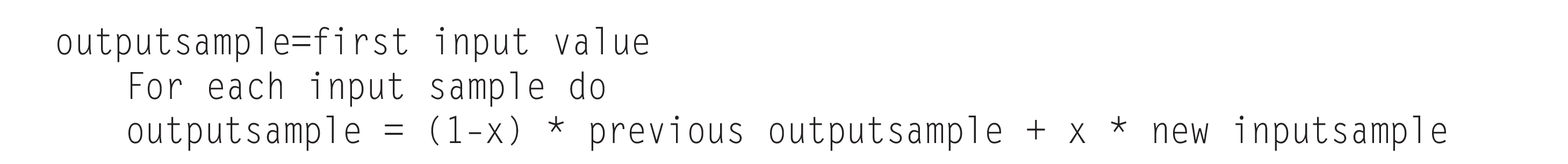Figure 3—Produce this algorithm by building a moving average filter by calculating the last 10 input values’ average.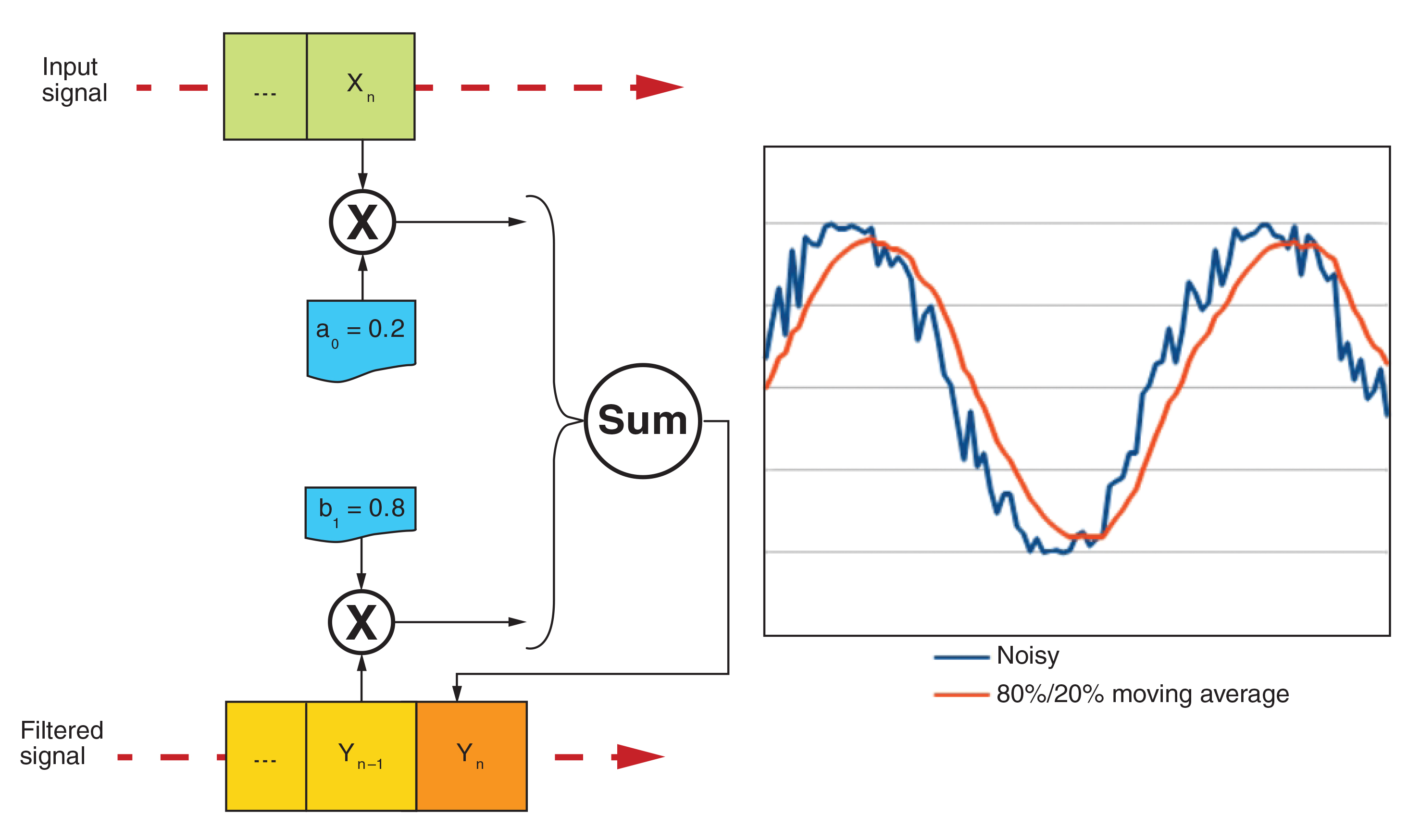Figure 4—A moving average filter is a basic example of an IIR filter. Here, the next output value is calculated as a weighted average of the input signal (20%) and previous output (80%). This implements a simple low-pass filter.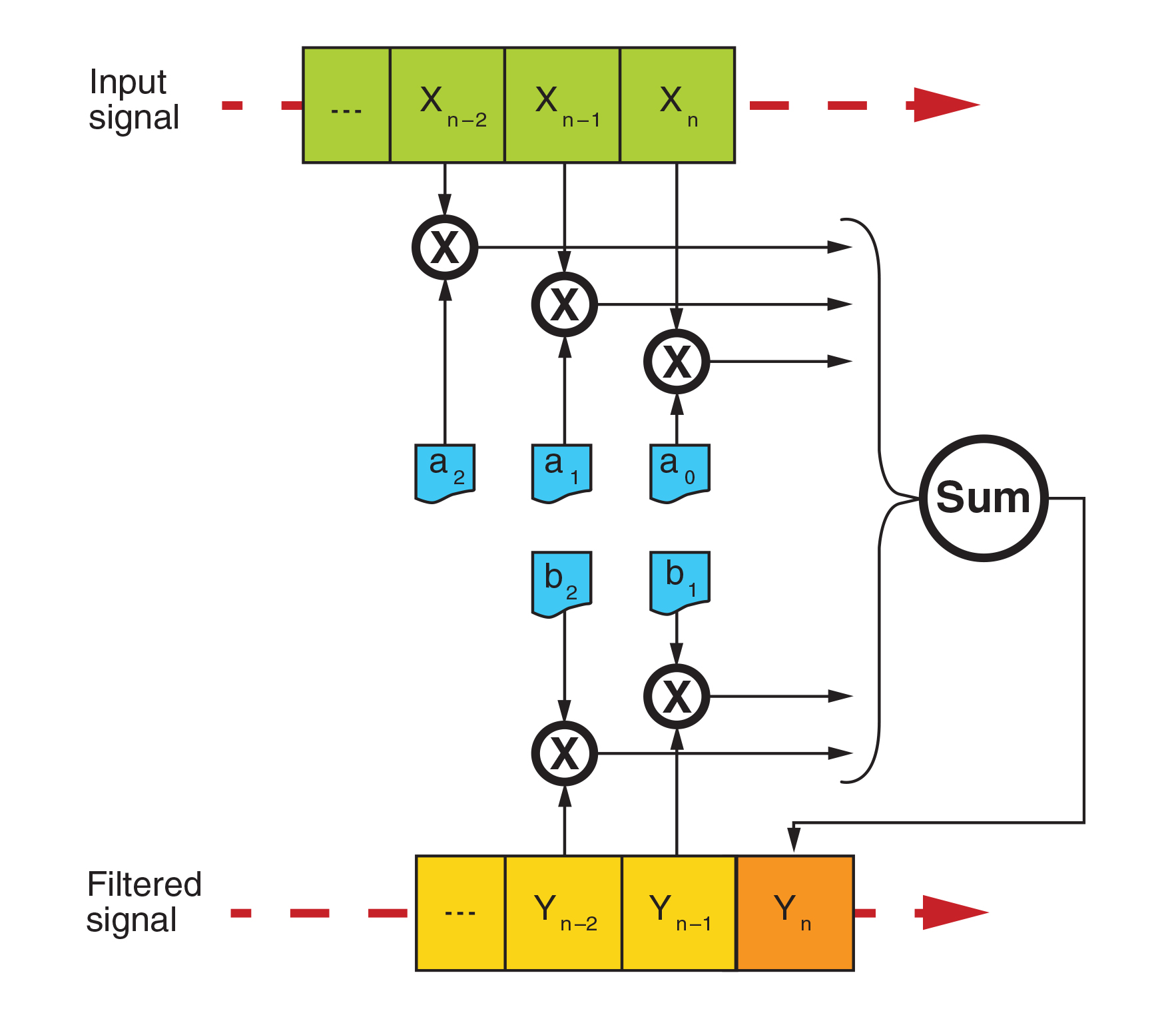Figure 5—Here is the generic form of an IIR filter. The next output value is a linear combination of the previous N input and output values. The number N is the filter’s rank, which is then defined by 2N-1 coefficients.
##### THE ANALOG WAY

As promised, I want to show you that an IIR filter is quite close to a classical analog filter. I will use the example of a basic low-pass RLC filter (see Figure 6). For this example, I selected a 1-MHz inductance and a 470-nF capacitor, which implies a resonant frequency of:

A small serial 22-Ω resistor attenuates this resonance a little, moving the 3-dB cutoff frequency up to around 11 kHz. I used Labcenter’s Proteus design software to create the simulation shown in Figure 6. The transient analysis shows a classic low-pass characteristic with a large overshoot, which could be easily reduced with a larger resistor. But that’s not my topic here, this is just an example. The corresponding simulation file is available on Circuit Cellar’s FTP site.

Now it’s time for some calculations. Assume you only have a spreadsheet and you are asked to calculate the output of this filter with a defined input signal, like in the transient simulation just executed. What would you do? First, draw a circuit diagram like the one shown in Figure 7, reopen your textbook, take a deep breath, and I’ll walk you through these simple calculations step by step. If you are really reluctant to use formulas, you could skip to the “real-world method” section, but you may miss something.Figure 6—Labcenter’s Proteus or any other SPICE-based simulator can be used to produce a simple RLC low-pass filter and its time and frequency domain simulation. Here the cutoff frequency is a little lower than 10 kHz.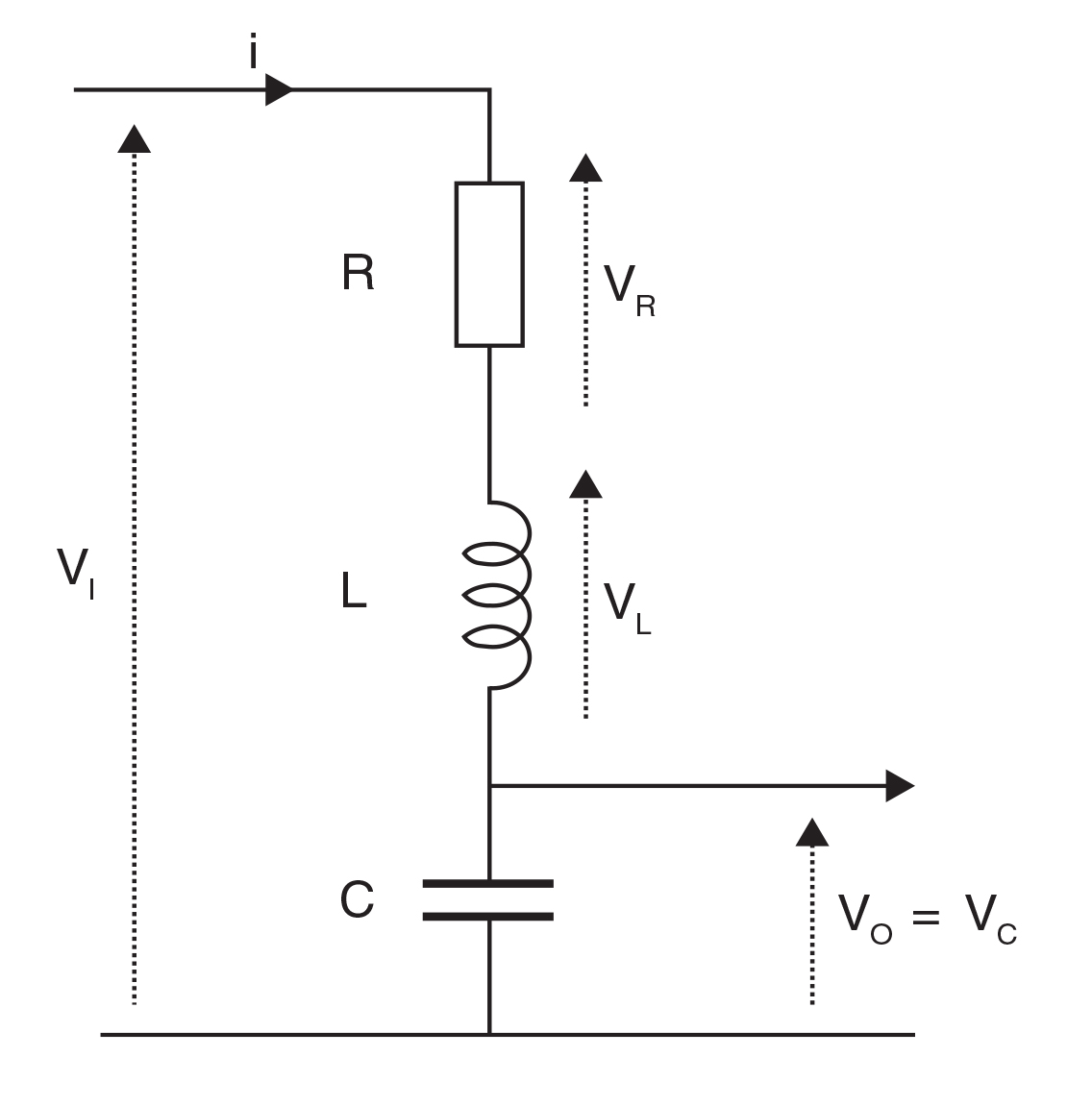Figure 7—A model of the RLC filter enables you to calculate the output voltage based on the input signal.

Let’s start with the basic equations. First, Kirchhoff’s voltage law says, the input voltage is the sum of the voltages across three parts:

Regarding the resistor, Ohm’s law says, the voltage across the resistor is proportional to the current:

Then, even if you may have forgotten, the two equations governing the inductor and capacitor are:

and

In these equations, the notation dx/dt means a derivative over time of the variable x, which means the slope of the curve x(t). The result of merging Equation 1, Equation 2, and Equation 3 is:

Let’s replace the current i by its value provided in Equation 4. The result is:

This is called a second-order partial derivative equation, and it is the fundamental equation governing this RLC circuit. The input voltage VI is linked to the output voltage VO but also to its first and second derivative over time. You will find plenty of textbooks explaining how to mathematically calculate its solutions. Depending on the parameter’s values (i.e., depending on the parts values), you will find either oscillating solutions or damped responses, as in any linear feedback system.

However, remember you have only a spreadsheet. Rather than trying to find an exact solution, take another approach, and try to approximate it with a discrete time-step method. The problem in this equation is the derivatives. But, if the time step is small compared to the signal variations’ speed, the derivative could be approximated over time of a function by the difference of two successive samples divided by the time step:

Similarly (trust me, or calculate it yourself), the second derivative can be approximated
by:

Using these approximations, you could rewrite Equation 6 without any derivatives. It’s a little boring, but I got :

Just shuffle around these terms and we will find how to calculate the next output value Vo(n) based on the other parameters :

which is a constant.

Here we are! Take another look at this formula. The next output sample is calculated as the last input sample VI(N) multiplied by a given constant, plus the previous output value VO (n – 1) × another constant, plus the previous output value VO (n – 2) also multiplied by a constant. Does it look familiar? Of course, this is exactly an IIR filter algorithm! I used The Document Foundation’s LibreOffice Calc to code this calculation on a spreadsheet (see Photo 1). Compare it with the plot in Figure 6, which should convince you that the calculation was correct. In fact, all circuit simulators (e.g., SPICE) use these types of discrete-time methods to internally do their calculations, so it is no surprise.Photo 1—Here is a spreadsheet version of the RLC filter transformed into an IIR filter. Look at the formula in the header bar. It is an exact transcription of the IIR calculation formula. The time-domain signal is, as expected, very close to the SPICE simulation.
##### REAL-WORLD METHOD

I have demonstrated how to construct an IIR filter from a standard analog filter. However, I’m sure you would not want to do this kind of calculation for a 12th-order bandpass filter. It would work, but it would require some long calculations.

Fortunately, there are solutions for the lazy engineer in the form of readyto- run IIR filter synthesis software. I know at least three free options. Refer to the Resources section.

The first solution is an online webbased IIR filter calculator developed by Tony Fisher from the University of York, England. You don’t need to install anything. You simply enter the type of filter you want (e.g., low pass, high pass, bandpass, or notch); select your preferred response (e.g., Bessel, Butterworth, or Chebyshev); enter the filter order, sampling frequency, and corner frequencies; and click on a browser button.

The second option is WinFilter software, named and developed by Adrian Kundert. Its last version is dated from 2004, but it still worked flawlessly on my Windows 7 PC and provided a great graphical interface. If you prefer opensource, generic-purpose software, you can also use SciLab Group’s SciLab, which I use quite often in projects featured in this column. SciLab is like a free version of MathWorks’s MATLAB, provided with a large library of signalprocessing functions, including IIR synthesis routines. In SciLab Group’s “Signal Processing with SciLab,” there is a 35-page chapter on IIR filters. If you are not constrained by free solutions, you can also find some great, inexpensive commercial software (e.g., Iowegian International’s ScopeIIR software or Fiwiz from Berkeley University’s International Computer Science Institute). I’ll use WinFilter as an example. Assume you are working on a 44-ksps digitized sound signal and you need to build a solid 3,000-Hz Butterworth lowpass filter to separate human voice from high-frequency noise or to reduce the sample rate. Imagine your system requires an attenuation of at least 20 dB at 6 kHz (i.e., one octave above the cutoff frequency). A first-order filter provides an attenuation of roughly 6 dB per octave, so you will need at least a fourth-order low-pass filter (e.g., 4 × 6 dB = 24 dB). So, you have your specifications. Now just download, install, and launch WinFilter, then enter the data. Click on Calculate Filter, and the magic happens. Within some hundreds of milliseconds, the tool will provide a simulated IIR filter that will fulfill your requirements and show you the simulated filter amplitude, phase response, impulse response, and group delay (see Photo 2). This is an invaluable tool.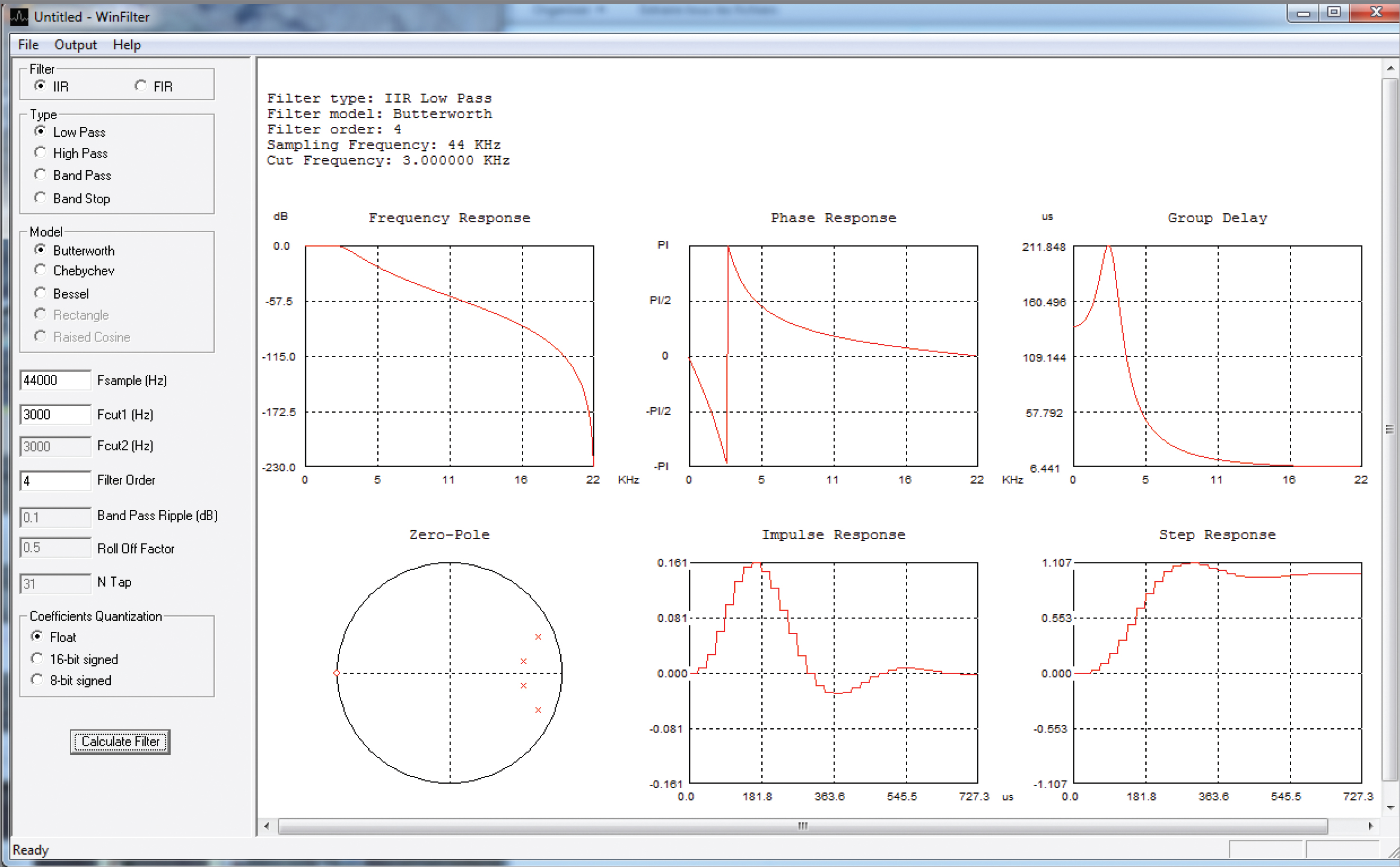Photo 2—WinFilter enables you to calculate and simulate all kinds of IIR filters by entering their key characteristics (left). The plots shows the resulting frequency and time behavior.

But the magic doesn’t stop there. Open the Output menu and you will find options to generate the corresponding C code for you, or even a VHDL version if you are FPGA addict. With WinFilter, there is the option to generate floating-point or integer code. The 16-bit integer version of the code generated for our 3,000-Hz low-pass filter is shown in Listing 1. This one will be easy to implement in any microcontroller using 32-bit arithmetic. However, before using such a filter in, say, a life-critical application, you’d better check its actual response. Using either an integer implementation or a fixedresolution floating-point version will inevitably imply truncation errors. And, if the filter is too critical (i.e., mathematically too close to the stability limit), unpleasant things may happen, as explained. This is difficult to anticipate without a closer look at the theory, but as a basic rule, the larger the pole number and the sharper the filter, the higher the risk. I must be honest. This is where IIR filters start to get complicated and nasty. A filter may work well with floating-point numbers and diverge when you port it to integers. This could be due to overflow errors but also due to truncation. Recursive algorithms such as IIR can sometimes be annoying.

``````Listing 1—For the low-pass example filter, I used WinFilter-generated C code with 16-bit integer coefficients.

/**************************************************************
WinFilter version 0.8
http://www.winfilter.20m.com
akundert@hotmail.com
Filter type: Low Pass
Filter model: Butterworth
Filter order: 4
Sampling Frequency: 44 KHz
Cut Frequency: 3.000000 KHz
Coefficents Quantization: 16-bit
Z domain Zeros
z = -1.000000 + j 0.000000
z = -1.000000 + j 0.000000
z = -1.000000 + j 0.000000
z = -1.000000 + j 0.000000
Z domain Poles
z = 0.657133 + j -0.115245
z = 0.657133 + j 0.115245
z = 0.785067 + j -0.331076
z = 0.785067 + j 0.331076
***************************************************************/
#define NCoef 4
#define DCgain 512
__int16 iir(__int16 NewSample) {
__int16 ACoef[NCoef+1] = {
5315,
21261,
31892,
21261,
5315
};
__int16 BCoef[NCoef+1] = {
8192,
-23629,
26498,
-13541,
2647
};
static __int32 y[NCoef+1]; //output samples
//Warning!!!!!! This variable should be signed (input sample width +
Coefs width + 4 )-bit width to avoid saturation.
static __int16 x[NCoef+1]; //input samples
int n;
//shift the old samples
for(n=NCoef; n>0; n—) {
x[n] = x[n-1];
y[n] = y[n-1];
}
//Calculate the new output
x = NewSample;
y = ACoef * x;
for(n=1; n<=NCoef; n++)
y += ACoef[n] * x[n] - BCoef[n] * y[n];
y /= BCoef;
return y / DCgain;
}``````

To check the filter’s behavior, I translated it into a SciLab code for you. The results are available on Circuit Cellar’s FTP site. The code simply generates a sine signal at increasing frequencies, passes it through the IIR filter, and plots the output amplitude based on frequency. The result, which is close to the expectation, is shown in Figure 8.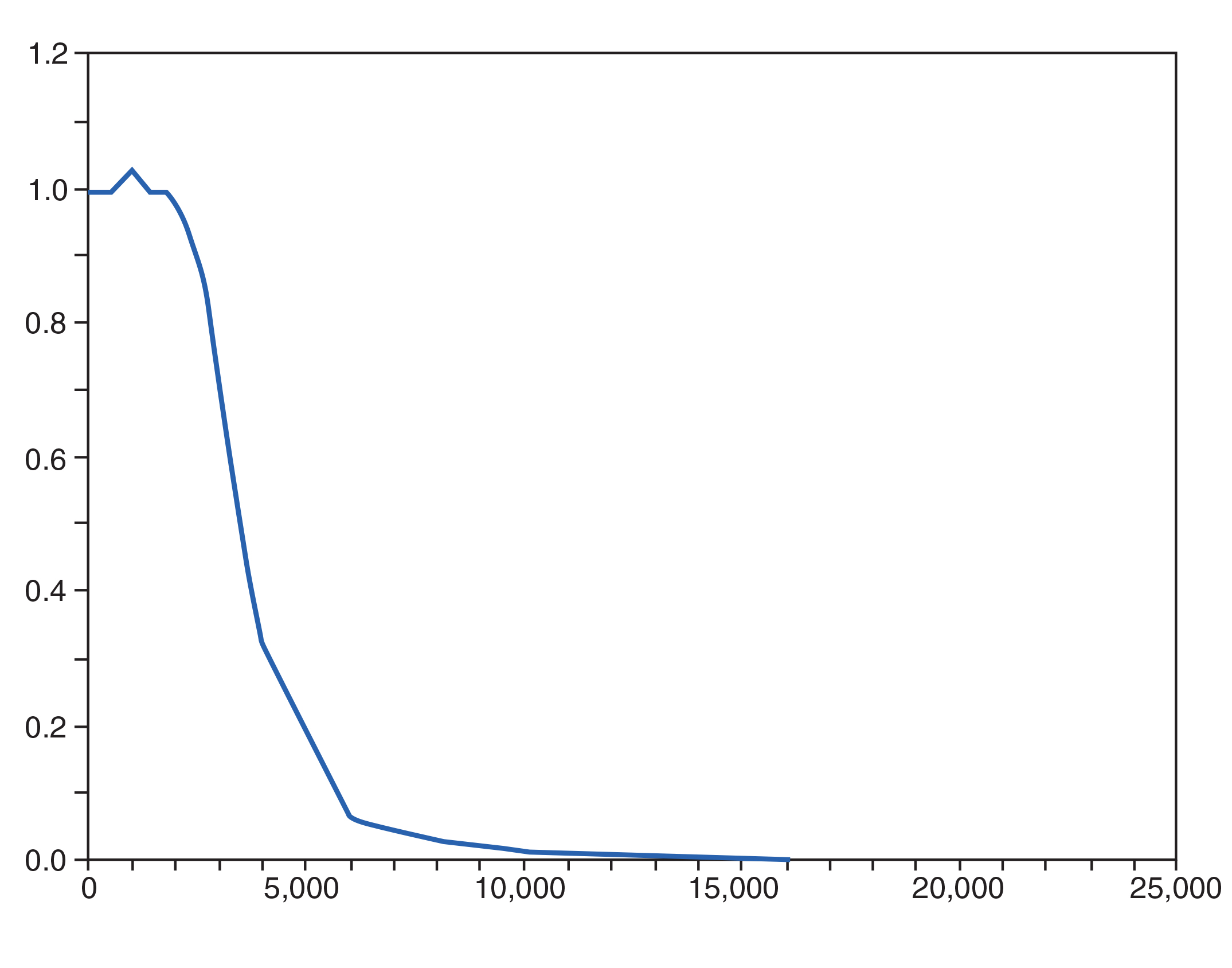Figure 8—The WinFilterbased filter was simulated with SciLab. This graph shows the output amplitude with varying signal frequency, which is close to what is expected.
##### WRAPPING UP

You can’t really understand a feedback system’s behavior—particularly IIR filters—without an actual mathematical analysis of the processing loop. I haven’t even explained the meaning of a pole and a zero. For complex filters, you will need to understand stability concerns and mathematical tools (e.g., Laplace transforms) to optimize them or even to design a solid system. However, as I’ve tried to show you in this article, designing a simple IIR filter and programming it in your application can be simple, thanks to the available tools. You should try. It will take one more technique out of your darker side! CC

Robert Lacoste lives near Paris, France. He has 23 years of experience working on embedded systems, analog designs, and wireless telecommunications. He has won prizes in more than 15 international design contests. In 2003, Robert started a consulting company, ALCIOM, to share his passion for innovative mixed-signal designs. You can reach him at rlacoste@alciom.com. Don’t forget to write “Darker Side” in the subject line to bypass his spam filters.

PROJECT FILES

RESOURCES
The Document Foundation, Libre Office Calc, www.documentfoundation.org.
Tony Fisher, “Interactive Digital Filter Design,” www-users.cs.york.ac.uk/~fisher/mkfilter.
The Scilab consortium, www.scilab.org
SciLab Group, “Signal Processing with SciLab,”http://wiki.scilab.org/Tutorials%20archivesaction=AttachFile&do=view&target=signal.pdf.

SOURCES
Fiwiz Digital filter design software
International Computer Science Institute (ICSI) | www1.icsi.berkeley.edu/~storn/fiwiz.html
ScopeIIR: IIR Filter design software for Windows
Iowegian International Corp. | www.iowegian.com
Proteus PCB design software
Labcenter Electronics, Ltd. | www.labcenter.com
MATLAB computing language
MathWorks | www.mathworks.com

PUBLISHED IN CIRCUIT CELLAR MAGAZINE • OCTOBER 2012 #267 – Get a PDF of the issue

 Keep up-to-date with our FREE Weekly Newsletter! Don't miss out on upcoming issues of Circuit Cellar. Subscribe to Circuit Cellar Magazine Note: We’ve made the May 2020 issue of Circuit Cellar available as a free sample issue. In it, you’ll find a rich variety of the kinds of articles and information that exemplify a typical issue of the current magazine. Would you like to write for Circuit Cellar? We are always accepting articles/posts from the technical community. Get in touch with us and let's discuss your ideas.